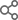【探讨】840D 换刀循环
2019-05-22 22:32:31

1）需要通过面板按钮进行主轴的高、低、空档切换，哪位有这方面的编程经验，请指教。

2）机床的刀库为链式伺服刀库，现在手动动作都测试完毕，如何实现自动M6 Txx换刀

3）自己编写了一段换刀宏程序，存放在用户循环目录下，现在执行换刀指令时，提示14011报警。【探讨】回复：840D 换刀循环
2019-08-22 08:33:40
12楼utt78：加我微信13478423520，备注西门子论坛。      2019-08-28 06:51:49
【探讨】回复：840D 换刀循环
2019-06-06 09:47:14
11楼utt78：PLC实现换刀的分步动作，然后子程序调用相应的分步动作      2019-08-21 08:08:40
【探讨】回复：840D 换刀循环
2019-05-25 07:58:43
10楼【探讨】回复：840D 换刀循环
2019-05-24 22:13:37
9楼【探讨】回复：840D 换刀循环
2019-05-24 07:48:34
8楼【探讨】回复：840D 换刀循环
2019-05-23 18:47:27
7楼

840D换刀可以通过下面几种方式：

1. 完全由PLC程序控制，子程序只是实现主轴定位和相关坐标轴定位；

2. 由换刀子程序和PLC结合来实现，可以参考《840Dsl刀库管理简明调试》；

3. 由换刀宏程序来实现；

上述几种方法都需要刀库管理来配合完成，可以找一下相关资料看一下。【探讨】回复：840D 换刀循环
2019-05-23 15:20:32
6楼【探讨】回复：840D 换刀循环
2019-05-23 09:52:15
5楼【探讨】回复：840D 换刀循环
2019-05-23 09:50:17
4楼

1. Txx代码激活刀库控制固定循环－MGZ。MGZ根据编程刀具号计算出该刀具所在的刀套位置，以及就近找刀的方向。位置和方向通过辅助功能H1（带符号的符点数）送到PLC。由PLC程序控制刀库按就近方向转动到编程刀具所在的位置，等待换刀。

2. M06启动换刀固定循环－TOOL。TOOL控制各坐标加入换刀位置，并启动机械手换刀动作。换刀完成后，激活刀具参数。

3. 边界条件：在程序参测试（V33000001.7=1）生效时，刀库和机械化手无换刀动作且编程的刀具参数生效。目的是在仿真时，刀库和机械手无动作，但刀具参数生效，以便仿真时可以检测软限位。

M06 ; 激活机械手换刀动作并且激活刀具参数；

2. 固定循环TOOL中，所有位移程序段应加G153（段有效）取消基本偏移。

3. 三个换刀过程：

4. 主轴准停角度利用PLC符点参数 MD14514: \$MN_USER_DATA_FLOAT

%_N_MGZ_SPF

;\$PATH=/_N_CST_DIR

PROC MGZ SAVE DISPLOF

;~~~~~~~~~~~~~~~~~~~~~~~~~~~~~~~~~~~~~~~~~~~~~~~~~~

; 前提条件:

; 初始化后, 刀具号与刀套号相等: 刀套号1 安装刀具T1

; 并且主轴刀套上无刀.

; - - - - - - - - - - - - - - - - - - - - - - - - -

; 参数说明:

; _TM:   当前刀库刀套号

; _TM:   编程刀具所在的刀套号

; _TM:   主轴刀套上安装的刀具号

; _TM:   1=程序测试标记

; _TM:   程序测试时主轴上的刀具号

; _ZSFR: 刀位差

; \$C_T:     编程刀具号

; \$PTOOLNO: 主轴刀套内的刀具号

; 刀具数据:

; \$TC_DP1[刀具号 ,1]: 刀具类型

; \$TC_DP3[刀具号 ,1]: 刀具几何参数-刀具长度1

; \$TC_DP6[刀具号 ,1]: 刀具几何参数-刀具半径

; \$TC_DP12[刀具号,1]: 刀具磨损- 长度1 方向

; \$TC_DP15[刀具号,1]: 刀具磨损- 半径  方向

; \$TC_DP24[刀具号,1]: 刀具尺寸0:正常 1: 过大

;                     在该子程序中表示空刀套

; \$TC_DP25[刀具号,1]: 刀套号

; - - - - - - - - - - - - - - - - - - - - - - - - -

; 程序结果:

; 通过辅助功能 H1 向 PLC发送编程刀具所在的刀套号以及

; 刀库的旋转方向 (方向通过 H1 的符号表示)

; 特殊情况:

; 在程序仿真和程序测试状态下无刀库和换刀动作. 为使程

; 序仿真时的刀具参数可以起作用, 本固定循环记录仿真前

; 主轴的有效刀具号, 在仿真结束时恢复主轴刀具, 然后再

; 进行换刀.

;~~~~~~~~~~~~~~~~~~~~~~~~~~~~~~~~~~~~~~~~~~~~~~~~~~

_TM=0                      ;跳转标志初始化

IF ((_TM==0)AND(\$P_ISTEST==1)) GOTOF _SIMU1

IF ((_TM==1)AND(\$P_ISTEST==1)) GOTOF _SIMU2

IF ((_TM==1)AND(\$P_ISTEST==0)) GOTOF _E_SIM

_ZSFR=\$MN_USER_DATA_INT  ;刀库最大刀位数

_ZSFR=_ZSFR/2            ;最大刀位数/2

IF \$C_T==\$P_TOOLNO GOTOF NOCHG1

IF \$C_T>_ZSFR   GOTOF NOCHG2;编程刀具> 最大刀具号

;~~~~~~~~~~~~~~~~~~~~~~~~~~~~~~~~~~~~~~~~~~~~~~~~~~

_T_CHG:

IF \$C_T<>0         GOTOF _T_N0

;T=0 时, 取出存储的空刀套号vvvvvvvvvvvvvvvvvvvvvvvv

_TM=_TM

GOTOF _T_POS

_T_N0:

MSG("编程刀具号为: T" <<\$C_T)

_TM=\$TC_DP25[\$C_T,1]

_T_POS:

;判断刀库旋转方向vvvvvvvvvvvvvvvvvvvvvvvvvvvvvvvvvv

_ZSFR=_TM-_TM           ;位置差

IF    _ZSFR==0  GOTOF _T_STEP

IF (((_ZSFR>0)AND(_ZSFR<=_ZSFR))OR((_ZSFR<0)AND(_ZSFR<-_ZSFR))) GOTOF _MGZ_P

IF (((_ZSFR>0)AND(_ZSFR>=_ZSFR))OR((_ZSFR<0)AND(_ZSFR>-_ZSFR))) GOTOF _MGZ_N

_MGZ_P:

H1=_TM

GOTOF _T_STEP

_MGZ_N:

H1=-_TM

;^^^^^^^^^^^^^^^^^^^^^^^^^^^^^^^^^^^^^^^^^^^^^^^^^^

_T_STEP:

;正常换刀时,T功能生效, 刀具参数在换刀后生效========

;R0=H1

T=\$C_T

;^^^^^^^^^^^^^^^^^^^^^^^^^^^^^^^^^^^^^^^^^^^^^^^^^^

GOTOF _END

_E_SIM:

;--------------------------------------------------

T=_TM                       ;恢复主轴刀具号

M96                            ;主轴刀具参数生效

MSG("恢复主轴刀具号:"<<_TM)

G04 F5

_TM=0                       ;清除程序测试标记

_TM=0                       ;

_TM=0                      ;

GOTOB _T_CHG

_SIMU1:

;仿真方式时,T功能生效, 刀具参数立即生效============

_TM=1                         ;记录程序测试状态

_TM=\$P_TOOLNO                 ;记录主轴刀具号

_TM=10                       ;无换刀动作

_SIMU2:

T=\$C_T

M96

;^^^^^^^^^^^^^^^^^^^^^^^^^^^^^^^^^^^^^^^^^^^^^^^^^^

GOTOF _END

NOCHG1:

_TM=10                       ;无换刀动作

MSG("没有换刀: 编程刀具等于主轴刀具                  ")

G04 F2

GOTOF _END

NOCHG2:

_TM=10                       ;无换刀动作

MSG("没有换刀: 编程刀号超过最大值. 循环启动继续或复位")

M00

_END:

M17

%_N_TOOL_SPF

;\$PATH=/_N_CST_DIR

PROC TOOL SAVE DISPLOF

IF _TM==10     GOTOF _END  ;没有找到刀具

;--------------------------------------------------

G500                           ;取消工件坐标系

D0                             ;取消刀补

; Z 轴返回固定点, 主轴准停, Mxx 启动机械手动作

M86 ;SPOS=\$MN_USER_DATA_FLOAT ;启动机械手换刀

;--------------------------------------------------

;

;

;

;--------------------------------------------------

IF \$C_T==0         GOTOF _RE_T ;还刀

IF \$P_TOOLNO==0    GOTOF _IN_T ;取刀

STOPRE

; 交换刀具 vvvvvvvvvvvvvvvvvvvvvvvvvvvvvvvvvvvvvvvv

\$TC_DP25[_TM,1]=_TM      ;编程刀具所在刀套号

\$TC_DP24[_TM,1]=0           ;清除主轴刀具标志

_TM=\$C_T                    ;主轴刀具套中为编程刀具

STOPRE

\$TC_DP25[_TM,1]=_TM      ;编程刀具所在刀套号

\$TC_DP24[_TM,1]=1           ;标出在主轴上的刀具

_TM=_TM

MSG("换刀步骤3:  换刀完成")

G04 F1

GOTOF _T_ACT

;~~~~~~~~~~~~~~~~~~~~~~~~~~~~~~~~~~~~~~~~~~~~~~~~~~

_IN_T:

; 装入刀具 vvvvvvvvvvvvvvvvvvvvvvvvvvvvvvvvvvvvvvvv

_TM=\$C_T                    ;主轴刀具套中为编程刀具

_TM=_TM                  ;刷新当前刀套号

_TM=_TM                  ;记忆首次取刀的刀套号

\$TC_DP24[_TM,1]=1           ;标出在主轴上的刀具

MSG("换刀步骤1:  装刀完成")

G04 F1

GOTOF _T_ACT

;~~~~~~~~~~~~~~~~~~~~~~~~~~~~~~~~~~~~~~~~~~~~~~~~~~

_RE_T:

; 退还刀具 vvvvvvvvvvvvvvvvvvvvvvvvvvvvvvvvvvvvvvvv

\$TC_DP24[_TM,1]=0           ;清除空刀套的位置

_TM=_TM                  ;主轴刀具返回空刀套

_TM=0                       ;主轴刀具套中刀具号为0

_TM=0                       ;主轴刀具套中刀具号为0

MSG("换刀步骤2:  还刀完成")

G04 F1

_T_ACT:

M96                            ;激活刀具参数

D1                             ;主轴恢复速度控制方式

_TM=_TM                  ;刷新当前刀套号

STOPRE

_END:

M17

%_N_INI_SPF

;\$PATH=/_N_CST_DIR

PROC INI SAVE DISPLOF

_TM=\$MN_USER_DATA_INT   ;刀库最大刀位数

_TM=1                       ;当前刀套号

_TM=0                       ;编程刀具所在刀套号

_TM=0                       ;主轴刀套中刀具号

_TM=0                       ;首次换刀的刀套号

_TM=0                       ;非程序测试状态

_TM=0                       ;程序测试时主轴刀具号

_TM=1                      ;计数器, 换刀步骤

_INI:

\$TC_DP25[_TM,1]=_TM    ;初始化刀套号

\$TC_DP24[_TM,1]=0          ;标出空刀套的位置

_TM=_TM+1

_TM=_TM-1

IF _TM > 0 GOTOB _INI

_TM=0

M99                            ;初始化PLC刀库计数器

T0

M96                            ;激活刀具参数

M17上一页 1/2    跳转> 下一页
© Siemens Ltd. China, 2018

×